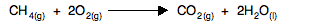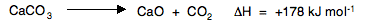AN INTRODUCTION TO GIBBS FREE ENERGY

```
```

This page introduces Gibbs free energy (often just called free energy), and shows how it can be used to predict the feasibility of reactions. If you have already read the page about how to do this with total entropy changes, you will find a little bit of repetition on this page.

```
```

Standard Gibbs free energy change, ΔG°

Calculating ΔG°

This is how standard Gibbs free energy change is calculated:

ΔG° = ΔH° - TΔS°

That's all you need to know. Learn it!

If you know (or can work out) the enthalpy change for the reaction, and you know (or can work out) the entropy change, and you know the temperature (in kelvin), then it would seem to be really easy to work out ΔG°.

There is an easy mistake to be made though! You have to remember that the entropy change is calculated in energy units of joules, but ΔG° and ΔH° are both measured in kJ.

Because you are calculating ΔG, your answer will be in kJ mol-1. You must remember to change the entropy change value into kJ before you start, otherwise you will get the calculation completely wrong.

On an earlier page in this section, we calculated the entropy change for the reactionΔS° worked out as -242.2 J K-1mol-1.

Before you do anything else, convert this to kJ by dividing by 1000.

ΔS° = -0.2422 kJ K-1mol-1.

This reaction is actually the combustion of methane, and so we can just take a value of this from a Data Book. But you should, of course, know how to calculate this from enthalpy changes of formation.

ΔH° = -890.4 kJ mol-1.

So if you had to calculate the Gibbs free energy change at, say, 298 K, you can just slot the numbers in:

ΔG° = ΔH° - TΔS°

ΔG° = -890.4 - 298(-0.2442) = -817.6 kJ mol-1

It is easy as long as you remember to convert the entropy change value into kJ.

```
```

Feasible (spontaneous) changes

In everyday life, something is spontaneous if it happens of its own accord, without any input from outside. The same thing is true in chemistry, but there is one major difference which defies everyday common sense.

If you drop marble chips (calcium carbonate) into dilute hydrochloric acid, there is an immediate fizzing. You don't need to do anything else - the reaction happens entirely of its own accord. It is a spontaneous change.

But in chemistry, a spontaneous change doesn't have to be rapid; in fact, it can be very, very, very slow indeed - even infinitely slow!

For example, carbon burns in oxygen to make carbon dioxide, but a piece of carbon will stay totally unchanged however long you keep it unless you first heat it. The energetics are right for a reaction to happen, but there is a huge activation energy.

Chemistry counts the reaction between carbon and oxygen as spontaneous! Personally, I think that is daft, and I prefer the word "feasible", which is often used in this topic. Throughout this topic I shall use the word "feasible", but if your examiners expect you to use the word "spontaneous", that is what you should do.

```
```

Feasible changes and ΔG

I am dropping the "standard" symbol after ΔG from now on, because most of the time we shall be using it at non-standard temperatures.

Whether or not a reaction (or other physical change) is feasible depends on the sign of ΔG. If ΔG is positive, then the reaction isn't feasible - it can't happen.

For a reaction to be feasible, ΔG has to be negative.

Remember that although it may be feasible, the reaction may not actually happen in any sensible time scale if there is a high activation energy barrier.

Note:  If your syllabus also wants you to work out feasibility in terms of ΔStotal, then beware that the the signs for feasibility are the opposite way around. So for a reaction to be feasible, ΔStotal has to be positive, but ΔG has to be negative.

This is potentially very confusing, and if you possible can, I suggest you stick to one method.

```
```

ΔG changes with temperature

Here is the important equation again:

ΔG = ΔH - TΔS

Note:  Remember that I have removed the standard symbols. We are no longer going to be talking about standard conditions, and so these don't apply any more. At this level, we always make the approximation that the values of ΔH and ΔS aren't affected by temperature.

Using this equation is easily illustrated using the decomposition of calcium carbonate:The value of ΔS for this reaction is +160.4 J K-1 mol-1.

Note:  In an exam, you may well be asked to calculate both the enthalpy change and the entropy change. Make sure that you can do that. If you find yourself values for enthalpies of formation, and for standard entropies, you should get answers similar to the ones I am using. Data sources vary, and so don't be worried if your answer is slightly different. If it is a lot different, then worry about it!

Remember that if you are using this in ΔG calculations, you first have to convert into kJ.

ΔS = +0.1604 kJ K-1 mol-1.

Suppose you had some calcium carbonate in the lab at 293 K. You can calculate a value of ΔG as:

ΔG = +178 - 293(+0.1604) = +131 kJ mol-1

The value is positive and so the reaction isn't feasible. It cannot happen at this temperature.

But suppose you heated it to 1000°C (1273 K). Recalculating gives:

ΔG = +178 - 1273(+0.1604) = -26.2 kJ mol-1

This value is negative, and so the reaction is feasible at this temperature. And you know, of course, that if you heat calcium carbonate strongly enough, it decomposes to give calcium oxide and carbon dioxide.

```
```

So how strongly do you have to heat it? You can work out an approximate temperature by finding out at what point ΔG becomes negative (i.e. less than 0).

Note:  It will only be an "approximate temperature" because of the approximations we make about ΔH and ΔS not changing with temperature. Don't worry about this at this level.

For a reaction to be feasible, the value of ΔG has to be less than 0. In mathematical terms, it is feasible if:

ΔG < 0

Because ΔG = ΔH - TΔS, that means that for a feasible reaction:

ΔH - TΔS < 0

If you know values for ΔH and ΔS, then you can work out a value for T which makes this expression less than 0.

In the case we are looking at

ΔH = +178 kJ mol-1, and

ΔS = +0.1604 kJ K-1 mol-1

Putting those values into the expression ΔH - TΔS < 0 gives

178 - T x 0.1604 < 0

You can treat the "less than" sign just like an equals sign, and so rearranging this gives:

178 < T x 0.1604

178 / 0.1604 < T

1110 < T

That's a strange way of looking at it, of course ("1110 is less than T."). But that is just the same as saying that T has to be greater than 1110 K.

```
```

Working out the effect of temperature without doing calculations

Look again at the equation:

ΔG = ΔH - TΔS

Remember that for a reaction to be feasible, ΔG has to be negative.

ΔH could be negative (an exothermic reaction) or positive (an endothermic reaction). Similarly ΔS could be either positive or negative.

There are four possible combinations of the signs of ΔH and ΔS. I want to look at those in turn.

```
```

Where ΔH is negative and ΔS is positive

In the equation ΔG = ΔH - TΔS:

ΔH is negative. TΔS is positive, and so -TΔS is negative.

Both terms are negative irrespective of the temperature, and so ΔG is also bound to be negative. The reaction will be feasible at all temperatures.

```
```

Where ΔH is positive and ΔS is negative

In the equation ΔG = ΔH - TΔS:

ΔH is positive. TΔS is negative, and so -TΔS is positive.

Both terms are positive irrespective of the temperature, and so ΔG is also bound to be positive. The reaction will not be feasible at any temperature.

```
```

Where ΔH is positive and ΔS is positive

In the equation ΔG = ΔH - TΔS:

ΔH is positive. TΔS is positive, and so -TΔS is negative.

Now increasing the temperature will change things. At higher temperatures, -TΔS will become more and more negative, and will eventually outweigh the effect of ΔH.

The reaction won't be feasible at low temperatures, but if you heat it, there will be a temperature at which it becomes feasible, because ΔG becomes negative.

The decomposition of calcium carbonate is a case like this, and we have done three calculations around it.

```
```

Where ΔH is negative and ΔS is negative

In the equation ΔG = ΔH - TΔS:

ΔH is negative. TΔS is negative, and so -TΔS is positive.

Again there will be a temperature effect. As temperature increases, -TΔS will become more and more positive, and will eventually outweigh the effect of ΔH.

At low temperatures, ΔG will be negative because of the effect of the negative ΔH, but as you increase the temperature, the effect of the positive -TΔS will eventually outweigh that. The value of ΔG will then become positive, and the reaction will no longer be feasible.

```
```

In summary

I really wouldn't suggest you tried to learn this - it is too confusing. Make sure that you understand it, so that when a question comes up you can work it out at the time.

You should where possible practise by finding questions from past papers from your examiners, checking them carefully against the mark schemes and examiner's reports if they are available. That way you will get a feel for what they think is important.

But it isn't too difficult to make up questions for yourself which would help you through the whole topic. Choose a simple reaction where you can find entropy values for everything involved. Use a data book if you have one, or find the equivalent information online, and avoid anything which involves solutions.

Use enthalpies of formation to work out the enthalpy change for the reaction. (If you use combustion reactions, for example, then you can just look up the enthalpy of combustion to save you the bother!)

Then work out the entropy change during the reaction. Decide what the effect of temperature will be on the feasibility of the reaction, and then work out values of ΔG at some widely different temperatures to see if you are right. (When you are doing those calculations, don't forget to correct the entropy change value from joules to kJ!)

```
```
 Questions to test your understanding Because this is all covered in detail in my calculations book I shan't be setting any questions throughout this section on entropy and free energy
```
```

Where would you like to go now?

To the entropy and free energy menu . . .

To the Physical Chemistry menu . . .

To Main Menu . . .

```
```